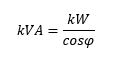FAQ’S

# What is the significance of the power factor in a generator?

Discover the different types of power kW, kVA, kVAr and its relation with the power factor (cosine of Fi (φ)).

To understand it, first, we will start by exploring the different types of power, on which the kW (kiloWatt – active power), the kVAr (kiloVolt Ampere reactive –reactive power) and the kVA (kiloVolt Ampere – apparent power) are electrical power measurements.

The kW is the power that generates work, kVAr is the used power that creates and maintains the electromagnetic fields of the inductive loads (engines, converters, ballast for discharge lamps, induction ovens) and the kVA is the product between a voltage (V) and a current (I), without reference to the phase angle.

The mathematic relation between the kVA and kW is the following:In this case, 0,8 is the value typically considered for the power factor in the three-phase gensets.

As a matter of fact, this value is only known if we measure the installation, because if the loads that the genset must feed, are essentially resistive (incandescent lamps, iron, oil heaters, among others) the value of kW≈kVA. But if we need to feed inductive loads, we may have power factor values close to 0,8. In some cases, we must use equipment’s to correct the power factor to acceptable values between 0,8 and 1.

The power factor is an indicator of energy efficiency, if it's close to 1, is synonymous of a more efficient installation, since it has less reactive energy (that doesn’t generate work) circulate in the circuit. If it's a low value, it indicates that the energy efficiency is low.

Find out the advantages of choosing a three-phase generator!